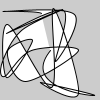# curve_vertices()#

Create a collection of curve vertices.

## Examples#import numpy as np

def setup():
random_curve_vertices = 100 * np.random.rand(25, 2)
py5.begin_shape()
py5.vertex(py5.width / 2, py5.height / 2)
py5.curve_vertices(random_curve_vertices)
py5.end_shape()


## Description#

Create a collection of curve vertices. The purpose of this method is to provide an alternative to repeatedly calling curve_vertex() in a loop. For a large number of curve vertices, the performance of curve_vertices() will be much faster.

The coordinates parameter should be a numpy array with one row for each curve vertex. There should be two or three columns for 2D or 3D points, respectively.

Underlying Processing method: curveVertices

## Signatures#

curve_vertices(
coordinates: npt.NDArray[np.floating],  # 2D array of curve vertex coordinates with 2 or 3 columns for 2D or 3D points, respectively
/,
) -> None


Updated on March 06, 2023 02:49:26am UTC